TitleGRE Math Practice Test II
"The Adaptive Test
P. 3 (Problems 20 - 28)

WTAMU > Virtual Math Lab > GRE MathIntroduction

 Because of the enormity of this part, I split the adaptive test into three pages.  P. 1 has the questions to all levels of the first 9 problems.  P. 2 has the questions to all levels of problems 10 - 19. P. 3 has the questions to all levels of problems 20 - 28. If you are looking at this page before P. 1, then you need to go to P. 1 of the adaptive test to start this adaptive test. Printing warning: Note that because of all of the levels per problem, there are a lot of questions on this page.  So if you wish to print this out, note that there will be a lot of pages to print.Practice Test II, Problems 20 - 28

 20 - 1.   Jill traveled 500 miles at a constant rate for 8 hours.  What was the constant rate that she was going?

 A)   64 mph  B)   61 mph  C)   65 mph  D)   60 mph  E)   62.5 mph

 20 - 2.   Courtney took a two day trip.  On the first day, she traveled 75 mph for 9 hours.  On the second day, she traveled 60 mph for 7 hours.  How far did she go on her two day trip?

 A)   675 miles  B)    420 miles  C)    255 miles  D)   1095 miles  E)    2000 miles

 20 - 3.   Overall, Carly went an average rate of 50 mph and it took her 8 hours to complete her journey.  If she traveled for 6 hours at 55 mph, what constant speed did she go for the remaining 2 hours?

 A) 52.5 mph  B) 50 mph  C) 55 mph  D) 35 mph  E) 45 mph

 20 - 4.   Jason took a two day trip.  On the first day, it took him 4 hours to travel 280 miles.  On the second day, it took him 6 hours to travel 390 miles.  What was Jason’s overall average rate?

 A) 70 mph  B) 65 mph  C) 67 mph  D) 67.5 mph  E) 68 mph

 20 - 5.   Sonny took a three day trip.  On the first day, it took him 10 hours to travel 500 miles.  On the second day it took him 8 hours to travel 480 miles.  On the third day, it took him 7 hours to travel 455 miles.  What was Sonny’s overall average rate?

 A) 50 mph  B) 60 mph  C) 65 mph  D) 58.3 mph  E) 57.4 mph

 21 - 1.   What is the area of a circle that is inscribed in a square whose area is 144 square centimeters?

 A)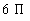square cm  B)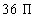square cm  C)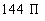square cm  D)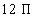square cm  E)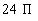square cm

 21 - 2.   What is the approximate area of a circle that is inscribed in a square whose area is 100 square centimeters?

 A)  314  square cm  B)   31.4  square cm  C)   62.8  square cm  D)   75     square cm  E)   78.5   square cm

 21 - 3.   What is the approximate area of a circle that is inscribed in a square whose area is 121 square centimeters?

 A) 95  square cm  B) 380  square cm  C) 69  square cm  D) 760  square cm  E) 100  square cm

 21 - 4.   What is the area of a square that is inscribed in a circle whose area is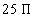square feet?

 A)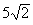square feet  B)     50  square feet  C)   312.5  square feet  D)   625  square feet  E)    25  square feet

 21 - 5.   What is the area of a square that is inscribed in a circle whose area is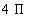square feet?

 A)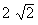square feet  B)    8  square feet  C)    4  square feet  D)    16  square feet  E)     2  square feet

 22 - 1.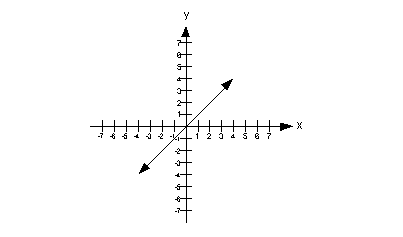Column A Slope of the line on graph above. Column B The x value of the x-intercept of the line on the graph above.

 A)  Column A’s quantity is greater.  B)  Column B’s quantity is greater.  C)  The quantities are the same.  D)  The relationship cannot be determined from the information given.

 22 - 2.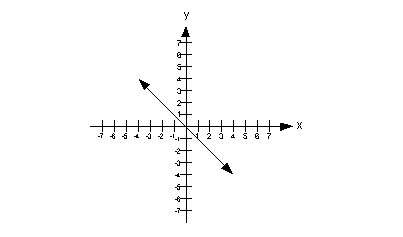Column A Slope of the line on graph above. Column B The x value of the x-intercept of the line on the graph above.

 A)  Column A’s quantity is greater.  B)  Column B’s quantity is greater.  C)  The quantities are the same.  D)  The relationship cannot be determined from the information given.

 22 - 3.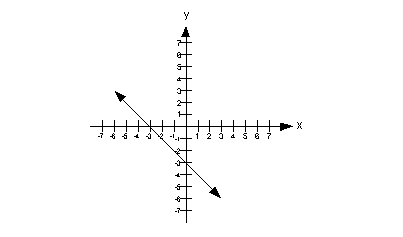Column A Slope of the line on graph above. Column B The x value of the x-intercept of the line on the graph above.

 A)  Column A’s quantity is greater.  B)  Column B’s quantity is greater.  C)  The quantities are the same.  D)  The relationship cannot be determined from the information given.

 22 - 4.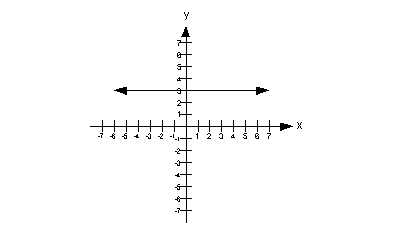Column A Slope of the line on graph above. Column B The y value of the y-intercept of the line on the graph above.

 A)  Column A’s quantity is greater.  B)  Column B’s quantity is greater.  C)  The quantities are the same.  D)  The relationship cannot be determined from the information given.

 22 - 5.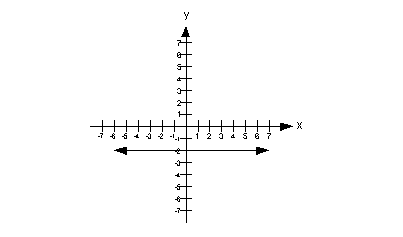Column A Slope of the line on graph above. Column B The y value of the y-intercept of the line on the graph above.

 A)  Column A’s quantity is greater.  B)  Column B’s quantity is greater.  C)  The quantities are the same.  D)  The relationship cannot be determined from the information given.

 23 - 1.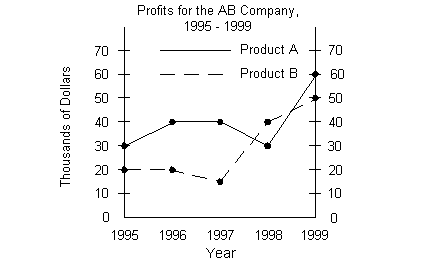Column A Difference of profits between Product A  and Product B in the year 1996. Column B The median of all of the profits for 1995 to 1999 for Product B.

 A)  Column A’s quantity is greater.  B)  Column B’s quantity is greater.  C)  The quantities are the same.  D)  The relationship cannot be determined from the information given.

 23 - 2.Column A Difference of profits between Product A and  Product B in the year 1996. Column B The mean of all of the profits for 1995 to 1999 for Product B.

 A)  Column A’s quantity is greater.  B)  Column B’s quantity is greater.  C)  The quantities are the same.  D)  The relationship cannot be determined from the information given.

 23 - 3.Column A The median of all of the profits for 1995 to  1999 for Product B. Column B The mean of all of the profits for 1995 to 1999 for Product B.

 A)  Column A’s quantity is greater.  B)  Column B’s quantity is greater.  C)  The quantities are the same.  D)  The relationship cannot be determined from the information given.

 23 - 4.Column A The mode of all of the profits for 1995 to  1999 for Product B. Column B The mean of all of the profits for 1995 to 1999 for Product B.

 A)  Column A’s quantity is greater.  B)  Column B’s quantity is greater.  C)  The quantities are the same.  D)  The relationship cannot be determined from the information given.

 23 - 5.Column A The mode of all of the profits for 1995 to 1999 for Product A. Column B The mean of all of the profits for 1995 to 1999 for Product A.

 A)  Column A’s quantity is greater.  B)  Column B’s quantity is greater.  C)  The quantities are the same.  D)  The relationship cannot be determined from the information given.

 24 - 1.For what year shown did the profits of Product A exceed the profits of Product B by the greatest number?

 A) 1995  B) 1996  C) 1997  D) 1998  E) 1999

 24 - 2.What was the total profits of Product B from 1995 to 1998?

 A)   \$200  B)   \$200,000  C)   \$145,000  D)   \$95  E)   \$95,000

 24 - 3.What was the difference in total profits between Product A from 1995 to 1999 and Product B from 1995 to 1999?

 A) \$55  B) \$550  C) \$5,500  D) \$55,000  E) \$550,000

 24 - 4.The percent increase in profits for Product A from 1995 to 1996 was approximately what %?

 A) 33%  B) 0%  C) 100%  D) 40%  E) 30%

 24 - 5.The percent decrease in profits for Product A from 1997 to 1998 was approximately what %?

 A) 25%  B) 0%  C) 20%  D) 30%  E) 35%

 25 - 1.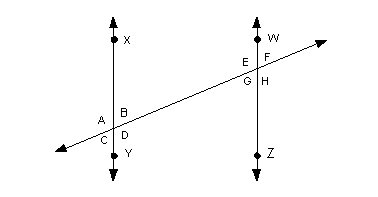Given that line XY is parallel to line WZ and angle A is 105 degrees, what is the measurement of angle H in degrees?

 A) 105  B)  75  C) 90  D) 180  E)  Not enough information is given to answer this question.

 25 - 2.Given that line XY is parallel to line WZ and angle A is 105 degrees, what is the measurement of the sum of angles C and  H in degrees?

 A) 105  B)  75  C) 90  D) 180  E)  Not enough information is given to answer this question.

 25 - 3.Given that line XY is parallel to line WZ and angle A is 105 degrees, what is the relationship between the measurements of angle F and angle D?

 A) They are equal to each other.  B) They sum up to be 90 degrees.  C) They sum up to be 105 degrees.  D) They sum up to be 180 degrees.  E)  Not enough information is given to answer this question.

 25 - 4.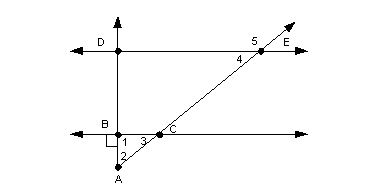Given that line DE is parallel to line BC, angle 2 is equal to angle 3, and angle 1 is a right angle, what is the measure of angle 5 in degrees?

 A) 45  B) 90  C) 135  D) 180  E)  Not enough information is given to answer this question.

 25 - 5.Given that line DE is parallel to line BC, angle 2 is equal to angle 3, and angle 1 is a right angle. What is the sum of the measures of angles 3, 4, and  5 in degrees?

 A) 45  B) 90  C) 180  D) 225  E)  Not enough information is given to answer this question.

 26 - 1.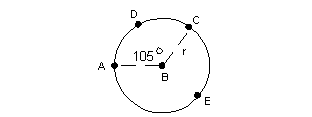If B is the center of the given circle above, what is the measurement in degrees of arc AEC?

 A) 90  B) 105  C) 250  D) 255  E)  Not enough information is given to answer this question.

 26 - 2.If B is the center of the given circle above, approximately what percent of the outer circle does arc ADC represent?

 A)  10%  B)  15%  C)  29%  D)  71%  E)   Not enough information is given to answer this question.

 26 - 3.If B is the center of the given circle above, approximately what percent of the outer circle does arc AEC represent?

 A)  10%  B)  15%  C)  29%  D)  71%  E)   Not enough information is given to answer this question.

 26 - 4.If B is the center of the given circle above and the radius is r, what is the circumference of arc ADC in radians?

 A)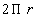B)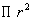C)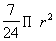D)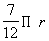E)    Not enough information is given to answer this question.

 26 - 5.If B is the center of the given circle above and the radius is r, what is the circumference of arc AEC in radians?

 A)B)C)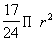D)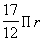E)    Not enough information is given to answer this question.

 27 - 1.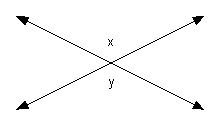Column A x Column B y

 A)  Column A’s quantity is greater.  B)  Column B’s quantity is greater.  C)  The quantities are the same.  D)  The relationship cannot be determined from the information given.

 27 - 2.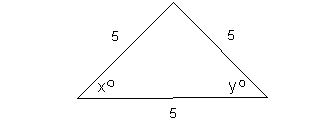Column A x Column B y

 A)  Column A’s quantity is greater.  B)  Column B’s quantity is greater.  C)  The quantities are the same.  D)  The relationship cannot be determined from the information given.

 27 - 3.

 Column A Number of sides of a octagon Column B Number of diagonals in a octagon

 A)  Column A’s quantity is greater.  B)  Column B’s quantity is greater.  C)  The quantities are the same.  D)  The relationship cannot be determined from the information given.

 27 - 4.   ABCD is a rectangle with width 5 and area 30.

 Column A Area of the rectangle Column B Perimeter of the rectangle

 A)  Column A’s quantity is greater.  B)  Column B’s quantity is greater.  C)  The quantities are the same.  D)  The relationship cannot be determined from the information given.

 27 - 5.   ABCD is a rectangle with length 4 and area 12.

 Column A Area of the rectangle Column B Perimeter of the rectangle

 A)  Column A’s quantity is greater.  B)  Column B’s quantity is greater.  C)  The quantities are the same.  D)  The relationship cannot be determined from the information given.

 28 - 1.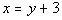and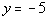Column A x Column B y

 A)  Column A’s quantity is greater.  B)  Column B’s quantity is greater.  C)  The quantities are the same.  D)  The relationship cannot be determined from the information given.

 28 - 2.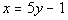and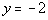Column A x Column B y

 A)  Column A’s quantity is greater.  B)  Column B’s quantity is greater.  C)  The quantities are the same.  D)  The relationship cannot be determined from the information given.

 28 - 3.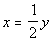and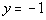Column A x Column B y

 A)  Column A’s quantity is greater.  B)  Column B’s quantity is greater.  C)  The quantities are the same.  D)  The relationship cannot be determined from the information given.

 28 - 4.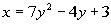andColumn A x Column B y

 A)  Column A’s quantity is greater.  B)  Column B’s quantity is greater.  C)  The quantities are the same.  D)  The relationship cannot be determined from the information given.

 28 - 5.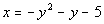andColumn A x Column B y

 A)  Column A’s quantity is greater.  B)  Column B’s quantity is greater.  C)  The quantities are the same.  D)  The relationship cannot be determined from the information given.

 You have finished the test!!!!  If you wish to have a quick check of your answer, go to the Answer Key Only link found below.  At this link you will find the answers to all of the levels of each question.  There are links to the Answer Key With Explanations, in case you missed a problem and have a question about it.  If you wish to have an explanation to each problem, go to the Answer Key With Explanations link found below.  When you get to this page it will have links to the mathematical area(s) that each question comes from in case you need to go back and review.

 Disclaimer: Note that we can not guarantee that you will pass your test after going through any of the tutorials in this website.   However, it will definitely help you to better understand the topics covered.  WTAMU and Kim Seward are not responsible for how a student does on any test for any reason including not being able to access the website due to any technology problems.  GRE and Graduate Record Examination are the registered trademarks of Educational Testing Service (ETS). The material here has neither been reviewed nor endorsed by ETS.  Throughout this website, we link to various outside sources.  WTAMU and Kim Seward do not have any ownership to any of these outside websites and cannot give you permission to make any kind of copies of anything  found at any of these websites that we link to.  It is purely for you to link to for information or fun as you go through the study session.  Each of these websites have a copy right clause that you need to read carefully if you are wanting to do anything other than go to the website and read it.  We discourage any illegal use of the webpages found at these sites.
 All contents copyright (C) 2003 - 2008, WTAMU and Kim Seward. All rights reserved.  Last revised on September 15, 2008 by Kim Seward.